# Simulating Automatic Climate Control Systems

This example shows how to simulate an automatic climate control system in a car using Simulink® and Stateflow®.

• In the User Setpoint in Celsius block, enter a value for the desired air temperature in the car.

• In the External Temperature in Celsius block, enter a value for the external air temperature.

• The Thermometer Display block indicates the reading of a temperature sensor placed behind the driver's head. This is the temperature that the driver feels.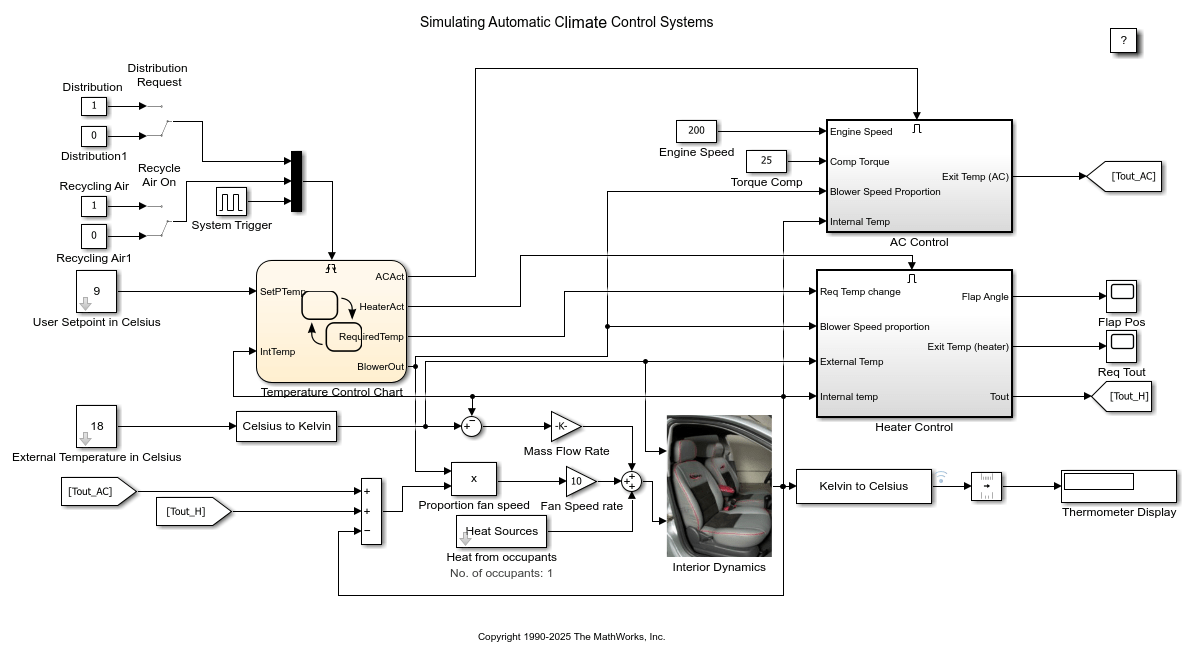Figure 1: The automatic climate control system

### Stateflow® Controller

Stateflow implements the supervisory controller. To see the control logic, open the chart.

The Heater_AC state shows that entering a setpoint temperature that is greater than the current car temperature by at least 0.5 deg C switches the heater system on. The heater remains active until the current temperature in the car is within 0.5 deg of the setpoint temperature. Similarly, when you enter a setpoint that is 0.5 deg C or less than the current car temperature, the air conditioner turns on. It stays active until the air temperature in the car is within 0.5 deg C of the setpoint temperature. To avoid continuously switching on and off the heater, the logic implements a dead band of 0.5 deg

In the Blower state, the greater the difference between the setpoint temperature and the current temperature, the harder the fan blows. This ensures that the temperature reaches the required value in a reasonable amount of time, despite the temperature difference. When the air temperature in the car is within 0.5 deg C of the setpoint temperature, the system switches off.

Two switches trigger the Stateflow charts that control the Air Distribution(AirDist) and Recycling Air (Recyc_Air) states. To facilitate effective window defrosting, the controller implements and an internal transition within these two states. When the defrost state is active, the controller turns off the recycling air.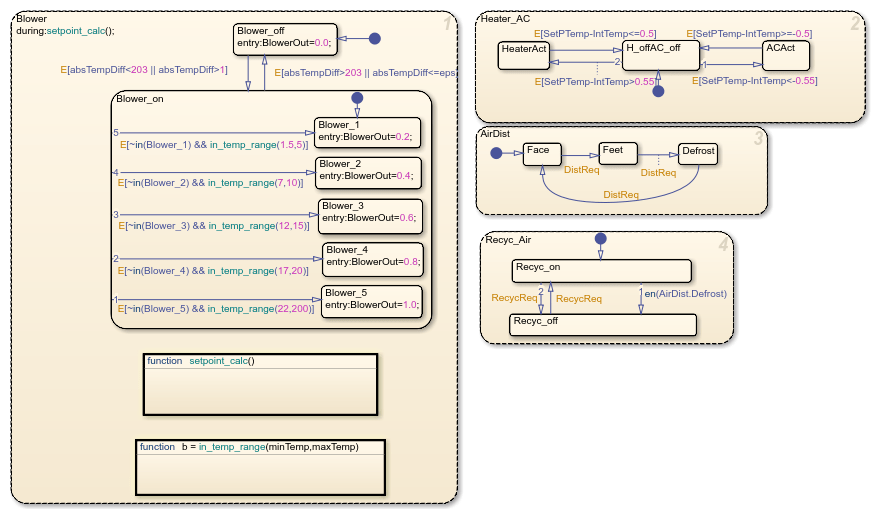Figure 2: The supervisory control logic in Stateflow

### Heater and Air Conditioner Models

The heater model implements this heat exchange equation:

`Tout = Ts - (Ts-Tin)e^[(-pi*D*L*hc)/(m_dot*Cp)]`

Where:

• Ts = constant (radiator wall temperature)

• D = 0.004m (channel diameter)

• L = 0.05m (radiator thickness)

• N = 30000 (Number of channels)

• k = 0.026 W/mK = constant (thermal conductivity of air)

• Cp = 1007 J/kgK = constant (specific heat of air)

• Laminar flow (hc = 3.66(k/D) = 23.8 W/m2K )

The models account for the heater flap. Similar to the blower operation, the greater the temperature difference between the required setpoint temperature and the current interior temperature, the greater the heating effect.

The air conditioner model implements this equation:

y*(w*Tcomp) = m_dot*(h4-h1)

Where:

• y = efficiency

• m_dot = mass flow rate

• w = speed of the engine

• Tcomp = compressor torque

• h4, h1 = enthalpy

The bang-bang control of the A/C system uses the engine speed and compressor torque to determine the temperature of the air that exits the A/C.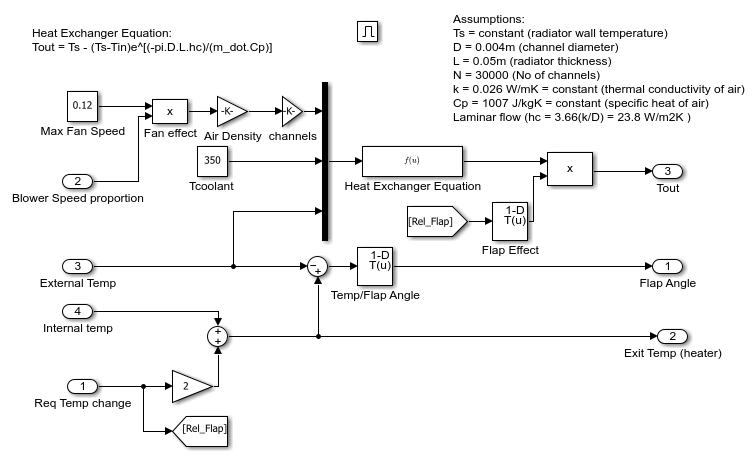Figure 3: Heater control subsystem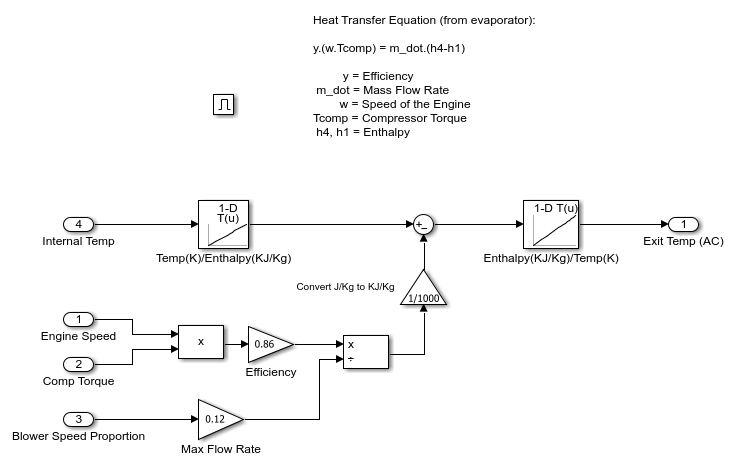Figure 4: A/C control subsystem

### Cabin Heat Transfer

These factors affect the temperature of the air felt by the driver:

• Temperature of the air exiting the vents

• Temperature of the outside air

• Number of people in the car

The factors are inputs into the thermodynamic model of the cabin interior. To account for the temperature of the air exiting the vents, the model calculates the difference between the vent air and the current car temperature and multiplies it by the fan speed proportion (mass flow rate). The model adds 100 W of energy is per person in the car. To account for air radiating into the car from the outside, the model multiplies the difference between the outside and interior air temperature by a smaller mass flow rate.

The Thermometer Display block displays the Interior Dynamics model output. It is a reading of a temperature sensor placed behind the driver's head. If you run the simulation with the default settings, the temperature reading starts at the external temperature of 18 °C and then cools to the user setpoint of 9 °C.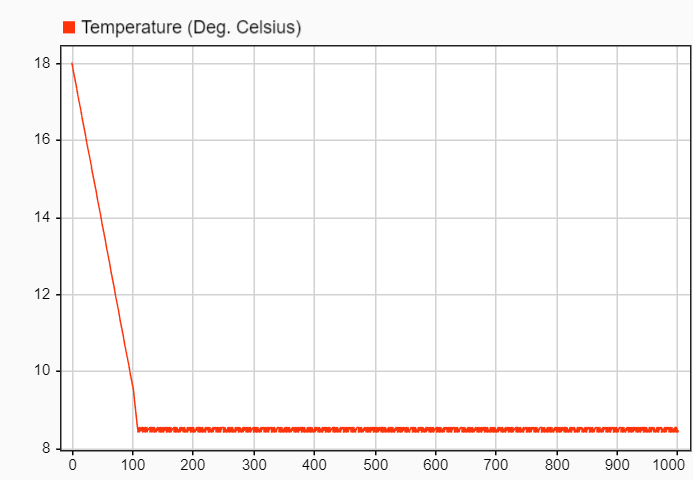Figure 5: Thermometer display versus time